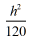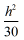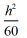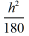Surveying And Analysis homework Help at TutorEye

# Best Homework Help For Surveying And Analysis

Our experts are available 24/7 to help you with Surveying And Analysis homework problems!

## Surveying And Analysis:

Surveying deals with the measurements and determination of the position of different points on the earth. It comprises several engineering operations, which includes assessment and evaluation of all land information.  It is to ensure that the construction of structures are positioned and built at the correct point relative to ground. Surveying determines relationships between different locations and derives all the information on the map for further study.

## Surveying And Analysis Sample Questions:

Question 1: Level surface is a curved surface at which each point is ________ to the direction of gravity at the point.

a) perpendicular                 b) parallel

c) coincidence                    d) none of these

Explanation:
Still the water surface is purely level surface.

Question 2: Which line is tangential to the level line and perpendicular to the plumb line?

a) Vertical line               b) Horizontal line

c) Datum line                d) None of these

Answer: b) Horizontal line

Explanation: It is the straight horizontal line tangential to the level

Question 3: A leminscate curve between the tangents will be transitional, if the polar deflection angle of its apex is

a) θ/2             b) θ/4

c) θ/3             d) θ/6

Explanation: For the curve to be transitional throughout, the maximum

Question 4: The desired sensitivity of bubble tubes with 3 mm divisions is 60. What is the radius of the bubble tube?

a) 12.11 mm             b) 11.10 mm

c) 10.31 mm             d) 13.21 mm

Answer: c) 10.31 mm

Explanation: Given,

Question 5: Which of the following methods is used to solve the three point problem?

a) Lehaman’s method              b) Bessel’s method

c) Tracing paper method          d) All of the above

Answer: d) All of the above

Explanation: The solution for the three point problem can be solved by the

Question 6: If a 60 m chain diverges through a perpendicular distance d from its correct alignment, the error in length, is

a)b)c)d)Answer: a)Explanation:  Length of the chain = L

Question 7: If the focal length of the object glass is 30 cm and the distance from the object glass to the trunnion axis is 60 cm, the additive constant is,

a) 0.3                b) 0.9

c) 0.6                d) 0.1

Explanation: f  = Focal length, d = Distance

Question 8: The Magnetic Bearing of a line is 48°24′. Calculate the true bearing if the magnetic declination is 5°38′ East.

a) 53°02′              b) 52°02′

c) 54°02′              d) 54°01′

Explanation: Magnetic Declination is the horizontal angle between true meridian and magnetic meridian.

Question 9: The __________ method consists in counting the number of paces between the two points of a line.

a) Contouring                b) Pacing

c) Leveling                    d) Chaining

Explanation: Pacing is a method of measurement using different chains.

Question 10: ___________ is a type of surveying, where the shape of the earth is taken into consideration.

a) Plane surveying                         b) Geodetic surveying

c) Hydrographic surveying             d) None of these

Answer: b) Geodetic surveying

Explanation: Geodetic surveying is the type of surveying in which